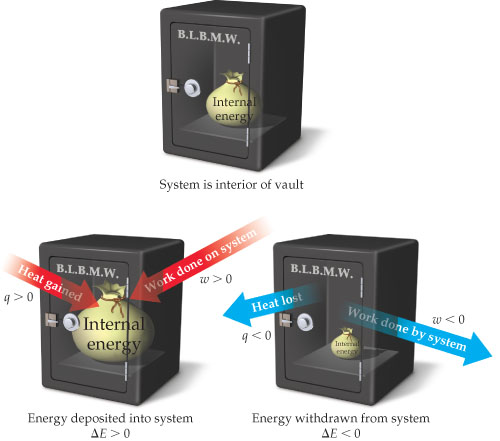# Problem: Sign conventions for heat and work. Heat, q, gained by a system and work, w, done on a system are both positive quantities, corresponding to "deposits" of internal energy into the system. Conversely, heat transferred from the system to the surroundings and work done by the system on the surroundings are both "withdrawals" of internal energy from the system.Suppose a system receives a “deposit” of 51 J of work from the surroundings and loses a “withdrawal” of 83 J of heat to the surroundings. What is the magnitude and the sign of E for this process?

###### FREE Expert Solution

We have to calculate the value of internal energy change (ΔE) for the process.

The internal energy(ΔE or ΔU) of a system can be calculated from the heat and work of the system.

The relationship between internal energy, heat, and work is shown in the following equation:

Where,

ΔE = internal energy

q = heat

w = work

98% (371 ratings)###### Problem DetailsSign conventions for heat and work. Heat, q, gained by a system and work, w, done on a system are both positive quantities, corresponding to "deposits" of internal energy into the system. Conversely, heat transferred from the system to the surroundings and work done by the system on the surroundings are both "withdrawals" of internal energy from the system.

Suppose a system receives a “deposit” of 51 J of work from the surroundings and loses a “withdrawal” of 83 J of heat to the surroundings. What is the magnitude and the sign of E for this process?